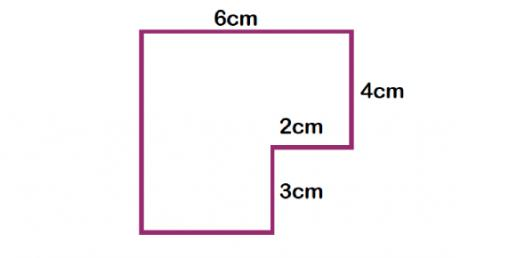# Perimeter

5 Questions | Total Attempts: 2857SettingsHere are a couple of practice questions that I would like you to do! Good Luck! Brandi

• 1.
If we have a triangle with the side lengths of 3, 4, 5, find the perimeter.
• A.

15

• B.

60

• C.

12

• D.

23

• 2.
If square has side lengths of 6 the perimeter will equal 24.
• A.

True

• B.

False

• 3.
A pentigon has a perimeter of 30 what must each side of the pentigon equal?
• 4.
Give the primeter of a rectangle with length 8 and width 4.
• A.

24

• B.

32

• C.

55

• D.

1

• 5.
If a triangle has sides with length 7 the perimeter will be 20.
• A.

True

• B.

False

Related TopicsBack to top